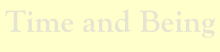Application.Music

Twelve-Tone Musical Scale

# of Semitones Interval Name Notes Consonant? Just Intonation* Equal Temperament Difference
0 unison C-C Yes 1/1=1.000 2^0/12=1.000 0.0%
1 semitone C-C# No 16/15=1.067 2^1/12=1.059 0.7%
2 whole tone C-D No 9/8=1.125 2^2/12=1.122 0.2%
3 minor third C-Eb Yes 6/5=1.200 2^3/12=1.189 0.9%
4 major third C-E Yes 5/4=1.250 2^4/12=1.260 0.8%
5 perfect fourth C-F Yes 4/3=1.333 2^5/12=1.335 0.1%
6 tritone C-F# No 7/5=1.400 2^6/12=1.414 1.0%
7 perfect fifth C-G Yes 3/2=1.500 2^7/12=1.498 0.1%
8 minor sixth C-Ab Yes 8/5=1.600 2^8/12=1.587 0.8%
9 major sixth C-A Yes 5/3=1.667 2^9/12=1.682 0.9%
10 minor seventh C-Bb No 9/5=1.800 2^10/12=1.782 1.0%
11 major seventh C-B No 15/8=1.875 2^11/12=1.888 0.7%
12 octave C-C' Yes 2/1=2.000 2^12/12=2.000 0.0%

* This table shows one variation of just intonation.

• Twelve-Tone Musical Scale. Why does our musical scale have twelve notes (counting both the white and black keys on the piano)? Why not ten or fifteen or twenty? The answer is: The twelve-tone equal-tempered scale is remarkable. The nearly perfect intervals seen in the table above are not typical of other equal-tempered scales. The twelve-tone equal-tempered scale is the smallest equal-tempered scale that contains all seven of the basic consonant intervals to a good approximation—within one percent. Furthermore, for the most important intervals, the fifth (3/2) and fourth (4/3), the approximations are better—within about one tenth of one percent.

Notes

Christian

• Cyber Hymnal. This site has over 3,800 Christian hymns and Gospel songs from many denominations. You’ll find lyrics, scores, MIDI files, pictures, history, and more.
• Digital Hymnal. Digital Hymnal, based on the Seventh-day Adventist Hymnal.
• SDA Hymnal.
• CCF Chord Book. Guitar chords for various Christian songs.
Classical
Other references Make a PVC Flute Southern Gospel Music